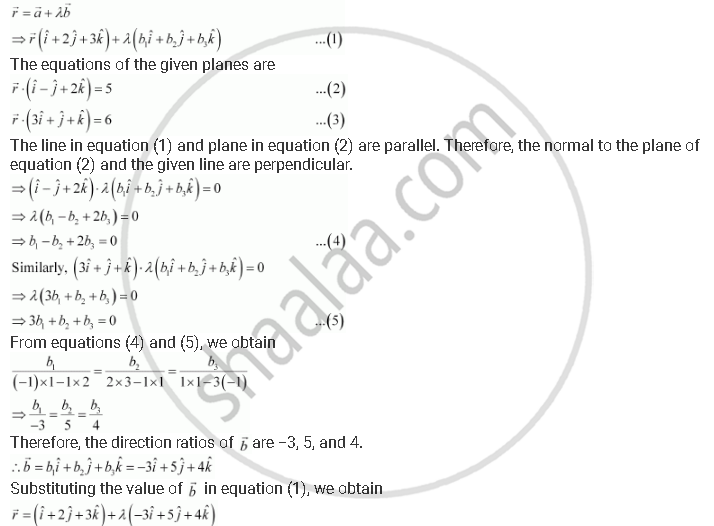Share

# Find the Vector Equation of the Line Passing Through (1, 2, 3) and Parallel to the Planes Vecr = (Hati - Hatj + 2hatk) = 5And Vecr.(3hati + Hatj + Hatk) = 6 - CBSE (Commerce) Class 12 - Mathematics

ConceptPlane Equation of a Plane Perpendicular to a Given Vector and Passing Through a Given Point

#### Question

Find the vector equation of the line passing through (1, 2, 3) and parallel to the planes vecr = (hati - hatj + 2hatk)  = 5and vecr.(3hati + hatj + hatk) = 6

#### Solution

Let the required line be parallel to vector vecb given by,

vecb = b_1hati + b_2hatj + b_3hatk

The position vector of the point (1, 2, 3) is veca = hati + 2hatj + 3hatk

The equation of line passing through (1, 2, 3) and parallel to vecbis given by,This is the equation of the required line.

Is there an error in this question or solution?

#### APPEARS IN

NCERT Solution for Mathematics Textbook for Class 12 (2018 to Current)
Chapter 11: Three Dimensional Geometry
Q: 19 | Page no. 499

#### Video TutorialsVIEW ALL 

Solution Find the Vector Equation of the Line Passing Through (1, 2, 3) and Parallel to the Planes Vecr = (Hati - Hatj + 2hatk) = 5And Vecr.(3hati + Hatj + Hatk) = 6 Concept: Plane - Equation of a Plane Perpendicular to a Given Vector and Passing Through a Given Point.
S